# Modulation Transfer Function

The modulation transfer function is the magnitude response of the optical system to sinusoids of different spatial frequencies. When we analyze an optical system in the frequency domain, we consider the imaging of sinewave inputs (Fig. 1.8) rather than point objects.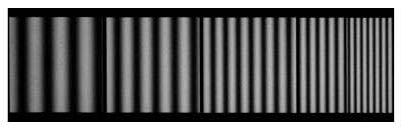Figure 1.8 Sinewave target of various spatial frequencies.

A linear shift-invariant optical system images a sinusoid as another sinusoid. The limited spatial resolution of the optical system results in a decrease in the modulation depth M of the image relative to what is was in the object distribution (Fig. 1.9). Modulation depth is defined as the amplitude of the irradiance variation divided by the bias level: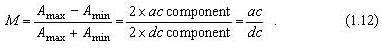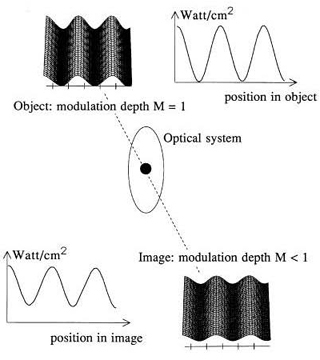Figure 1.9 Modulation depth decreases going from object to image.

We can see from Fig. 1.10 that modulation depth is a measure of contrast, with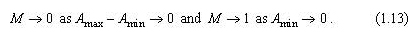The M = 0 condition means that, although there is still a nonzero image-irradiance level, there is no spatial variation of that irradiance. When the waveform has a minimum value of zero, there is unit modulation depth—whatever the maximum irradiance level is. Low levels of modulation depth are harder to discern against the unavoidable levels of noise inherent in any practical system.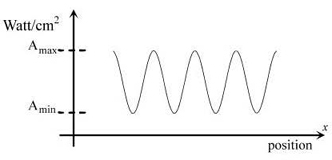Figure 1.10 Definition of modulation depth.

The finite size of the impulse response of the optical system causes a filling in of the valleys of the sinusoid and a lowering of the peak levels. The effect of this is to decrease the modulation depth in the image relative to that in the object. Defining the modulation transfer (MT) as the ratio of modulation in the image to that in the object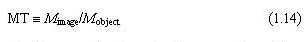we find that the reduction of modulation transfer is spatial-frequency dependent. The limited resolution of the optics is more important at high spatial frequencies, where the scale of the detail is smaller. As seen in Fig. 1.11, when we plot modulation transfer against spatial frequency, we obtain the MTF, generally a decreasing function of spatial frequency. The MTF is the image modulation as a function of spatial frequency (assuming a constant object modulation),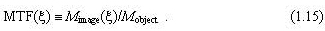The M of the object waveform does not need to be unity—if a lower input modulation is used, then the image modulation will be proportionally lower.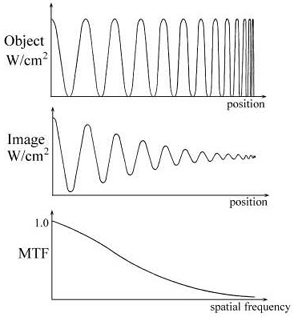Figure 1.11 Modulation transfer function is the decrease of modulation depth with increasing frequency.
Citation:

G. D. Boreman, Modulation Transfer Function in Optical and Electro-Optical Systems, SPIE Press, Bellingham, WA (2001).